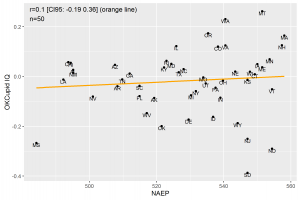In the interest of publishing null findings: I tried estimating US state IQs from the mean cognitive ability for users in the OKCupid dataset. However, this did not work out. This was a far shot to begin with due to massive self-selection and somewhat non-random sampling.

Actually, what I really wanted was another way to estimate county-level IQs, since Add Health refuses to share that data. But before I could do that, I needed to validate the estimates for something else. The scatterplot can be seen below. The NAEP is from Admixture in the Americas, so it is based on a few years of NAEP data.R code

This assumes you have loaded the OKCupid data as d_main and have already calculated the cognitive ability scores.

```### VALIDATE STATE-LEVEL IQs

# subset data -------------------------------------------------------------
v_2chars = d_main\$d_country %>% str_length() < 3
v_notUK = !d_main\$d_country %in% c("UK", "GU", "13", NA)
d_states = d_main[v_2chars & v_notUK, ]

#mean score by
d_states = ddply(d_states, .(d_country), .fun = plyr::summarize, IQ = mean(CA, na.rm = T))
rownames(d_states) = d_states\$d_country